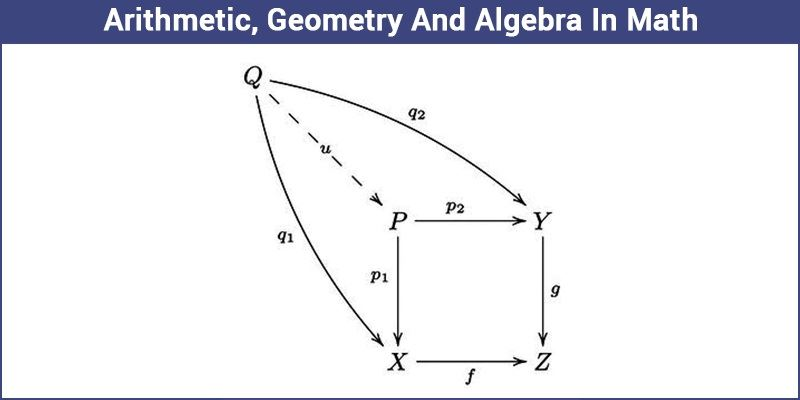# Basics of Mathematics: Arithmetic, Geometry, Algebra & Words

The first thing that you should know about Mathematics is that it is not a subject, it is a language. Math is a language that you can learn just like English, French, Hindi and all the others. There are basically 4 components of this language which are Arithmetic, Geometry, Algebra, and words. You can represent everything that you learn in Math using one of these 4 components:## Basics of Mathematics

Here, we are going to discuss the four basic components of Mathematics here in detail with examples. Go through the below article and get to know about the uses of these components.

### Arithmetic – Numbers and Operations

This is where Mathematics began, the first method of counting was counting on fingers. This evolved into sign language for the hand-to-eye communication of numbers. Tallies made by carving notches in wood, bone, and stone were used for at least forty thousand years, post this we started to count. Then came numbers.

The branch of mathematics dealing with the properties and manipulation of numbers is called Arithmetic. You are already familiar with all the arithmetic operations: Addition (+), Subtraction (-), Multiplication (*) and Division (/). Other concepts like powers, roots, logs, exponents etc.exist but all of them are just extensions to the initial 4 arithmetic operations.

### Geometry – Shapes

Mathematically, what came into existence in the very beginning were numbers drawn as diagrams or diagrams represented as numbers. These are triangles,  squares,  rectangles,  circles, ellipses and all other different shapes and diagrams. This brings us to a branch called Geometry. Euclid, the Father of Geometry, believed that Geometry is what was used by God to create nature.

### Algebra – Expressions and Equations

The branch of mathematics which deals with the format of representation of variables is what we call as Algebra. It includes various algebraic expressions and equations. Algebra is one of the branches of Mathematics, which is used by everyone in real life.

### Words – Mathematical Statement

To bring some order to all the chaos created, we have words to explain the above mathematical concepts. In a simple line, what is mathematics? It’s just about conversion of relations from one form to another, according to four ideas are given above.